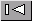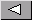Math Central - mathcentral.uregina.caResource RoomResource RoomStrandSecondary - Equations and Problem Solvingstart over

We found 34 items matching your search.Page3/3In this note Penny discuss the use of row operations on matrices to solve systems of linear equations and show how this idea is used to find the inverse of a square matrix. AUTHOR(S): Penny NomAn approach to teaching permutations and combinations that leads to the Binomial Theorem. AUTHOR(S): Penny NomStudents often ask where they will ever use trigonometry or why they need to study it. This note contains a use of trigonomerty in two areas where its use might be unexpected. One situation involves an artisan who needs the dimensions for a metal sculpture he is constructing and the second a homeowner who is disputing her property assessment. AUTHOR(S): Penny NomDans l'exemple ci-dessous, nous partons d'une situation familière è l'élève pour arriver au modèle mathématique. Un des avantages de cette activité est qu'il n'y a pas de solution unique. Trouver diverses AUTHOR(S): Denis and Diane HansonPage3/3Math Central is supported by the University of Regina and The Pacific Institute for the Mathematical Sciences.about math central :: site map :: links :: notre site français